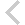FICO Xpress Optimization Examples Repository
 FICO Optimization Community FICO Xpress Optimization HomeProject scheduling problem with disjunctive resource constraints

Description
Project scheduling problem with disjunctive resource constraints and minimum and maximum delays between tasks, formulated
• with 'disjunctive' constraints (bridgescheduling.mos), or
• using task and resources objects (bridgescheduling_alt.mos)

Source Files
By clicking on a file name, a preview is opened at the bottom of this page.

Data Files

bridgescheduling.mos

(!****************************************************************

CP example problems
===================

file bridgescheduling.mos

Project scheduling problem with disjunctive resource constraints.

We wish to schedule a set of tasks for the construction of a
bridge. Each task uses a particular resource (excavator,
pileDriver, ...) and can only start after the completion of its
predecessor(s). A resource can only be used by one task at a
time (disjunctive resources).
The aim is to minimize the total duration of the schedule (PE).

*** This model cannot be run with a Community Licence
for the provided data instance ***

Copyright(C) 2008 Artelys S.A. and Fair Isaac Corporation
Creation: 2005, rev. Apr. 2022
*****************************************************************!)

model "Bridge Scheduling (CP)"
uses "kalis"

declarations
RESOURCES: set of string            ! Set of resources

SetEE, SetES, SetSS, SetSE: range   ! Index sets of distance data
DISTEE: array(SetEE,1..2) of string ! End-to-end max. distance
DISTES: array(SetES,1..2) of string ! End-to-start max. distance
DISTSS: array(SetSS,1..2) of string ! Start-to-start min. distance
DISTSE: array(SetSE,1..2) of string ! Start-to-end min. distance
end-declarations

initializations from "Data/bridgescheduling.dat"
PRECS
DISTEE DISTES DISTSS DISTSE
end-initializations

forall(t in TASKS | USE(t)<>"") RESOURCES += {USE(t)}

setparam("KALIS_DEFAULT_LB", 0)

declarations
Disj,Disj_crane,Disj_brick: set of cpctr  ! Disjunction constraints
Strategy: array(range) of cpbranching     ! Branching strategy
end-declarations

!******************************************************************
! **** Create disjunctions between tasks using the same resource ****
procedure disjunctive_constraints(r:string)
declarations
usedby: set of cpvar
durby : array(cpvar) of integer
end-declarations

nbr := 0
forall (t in TASKS | USE(t) = r) do
usedby += {start(t)}
durby(start(t)) := DUR(t)
nbr += 1
end-do
if (r="crane") then
disjunctive(usedby,durby,Disj_crane,0)
elif (r="bricklaying") then
disjunctive(usedby,durby,Disj_brick,0)
else
disjunctive(usedby,durby,Disj,0)
end-if
end-procedure

! **** Distance constraints between starts or ends of certain task pairs ****
procedure distance_constraints
forall(i in SetEE)                    ! End-to-end max. distance
start(DISTEE(i,1)) + DUR(DISTEE(i,1)) + 4 >= start(DISTEE(i,2)) +
DUR(DISTEE(i,2))
forall(i in SetES)                    ! End-to-start max. distance
start(DISTES(i,1)) + DUR(DISTES(i,1)) + 3 >= start(DISTES(i,2))
forall(i in SetSS)                    ! Start-to-start min. distance
start(DISTSS(i,1)) + 6 <= start(DISTSS(i,2))
forall(i in SetSE)                    ! Start-to-end min. distance
start(DISTSE(i,1)) + DUR(DISTSE(i,1)) - 2 <= start(DISTSE(i,2))
end-procedure

!******************************************************************
! **** Model definition *****
setparam("kalis_auto_propagate", false) ! Do not post constraints at definition

forall(t in TASKS) setname(start(t),t) ! Set variable names

! Precedences between a task and its predecessors
forall(t in TASKS, i in 1..NPREC(t))
start(PRECS(t,i)) + DUR(PRECS(t,i)) <= start(t)

! Disjunctions between tasks using the same resource
forall(r in RESOURCES) disjunctive_constraints(r)

! Define min/max distance between certain starts and ends of task pairs
distance_constraints

! Post all constraints
if not cp_propagate then
writeln("Problem is infeasible")
exit(1)
end-if

! Define the branching strategy: branch first on bottleneck resources
Strategy(1) := settle_disjunction(Disj_crane)
Strategy(2) := settle_disjunction(Disj_brick)
Strategy(3) := split_domain(KALIS_LARGEST_MAX, KALIS_MIN_TO_MAX)
cp_set_branching(Strategy)

! Uncomment to limit computation time
setparam("KALIS_MAX_COMPUTATION_TIME", 10)

setparam("KALIS_VERBOSE_LEVEL", 1)

if not cp_minimize(start("PE")) then
writeln("Problem is infeasible")
exit(1)
end-if

ct:= 0
write(t, "=", getsol(start(t)), if(ct mod 10=9, ",\n", ", "))
ct+=1
end-do
writeln("\nMakespan: ", getsol(start("PE")))

writeln("Proof of optimality in:")
cp_show_stats

end-model

`